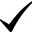# Course Schedule

Course Schedule

## Course Schedule

Course
Course Description
Fall
Spring
MATH 1350
Calculus I

Selected topics in analytical geometry and calculus. Rates of change of functions, limits, derivatives of algebraic and transcendental functions, applications of derivatives, and integration.

Prerequisite: Satisfactory completion of a course similar to Precalculus (MATH 1050) *** See Mathematics Department prerequisite policy. ***

4 Credit HoursMATH 1360
Calculus II

Continuation of Calculus I (MATH 1350). Transcendental functions, techniques and applications of integration, Taylor's theorem, improper integrals, infinite series, analytic geometry, polar coordinates, introduction to differential equations.

Prerequisite: Satisfactory completion of a course similar to Calculus I (MATH 1350), or a score of 3 or more on the AP Calculus AB or BC exam.

4 Credit Hours*
MATH 2350
Calculus III

Continuation of Calculus II (MATH 1360). Parametric curves, vector functions, partial differentiation, multiple integrals, Green's Theorem and Stoke's Theorem.

Prerequisite: Satisfactory completion of a course similar to Calculus II (MATH 1360), or a score of 4 or more on the AP Calculus BC exam.

4 Credit Hours*
MATH 2150
Discrete Mathematics

Introduction to most of the important topics of discrete mathematics, including set theory, logic, number theory, recursion, combinatorics, and graph theory. Much emphasis will be focused on the ideas and methods of mathematical proofs, including induction and contradiction.

Prerequisite: Satisfactory completion of at least one semester of calculus.

3 Credit HoursMATH 3110
Theory of Numbers

A careful study, with emphasis on proofs, of the following topics associated with the set of integers: divisibility, congruences, arithmetic functions, sums of squares, quadratic residues and reciprocity, and elementary results on distributions of primes.

Prerequisite: MATH 2150 (Discrete Mathematics), and either MATH 1360 (Calculus 2) or score of 4 or 5 on the AP Calculus AB or BC exam.

3 Credit HoursMATH 3130
Intro to Linear Algebra

Systems of linear equations, matrices, vector spaces, linear independence, basis dimension, determinants, linear transformations and matrices, eigenvalues and eigenvectors.

Prerequisite: Completion of or concurrent enrollment in Multivariable calculus course (equivalent of Math 2350, Calculus III)

3 Credit HoursMATH 3400
Intro to Differential Equations

First order differential equations, linear second order differential equations, the Laplace transform method, power series solutions, numerical solutions, and linear systems.

Prerequisite: Satisfactory completion of at least two semesters of calculus, including the study of series and sequences and improper integrals.

3 Credit HoursMATH 3410
Intro to Analysis

An introduction to proofs in analysis. Topics include completeness of the real numbers, sequences and limits, infinite series, and continuous functions. Prer., MATH 2150 and MATH 2350.

Prerequisite:  MATH 2150, Discrete Mathematics and MATH 2350, Calculus III.

3 Credit HoursMATH 3810
Intro to Probability and Stats

The axioms of probability and conditional probability will be studied as well as the development, applications and simulation of discrete and continuous probability distributions. Also, expectation, variance, correlation, sum and joint distributions of random variables will be studied. The Law of Large Numbers and the Central Limit Theorem will be developed. Applications to statistics will include regression, confidence intervals, and hypothesis testing.

Prerequisite: satisfactory completion of a Multivariable calculus course (equivalent of Math 2350, Calculus III).

3 Credit Hours* - Course offered as needed